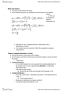# ECO220Y1 Lecture Notes - Lecture 19: Confidence Interval, Null Hypothesis, Prediction Interval

24 views2 pages
School
Department
Coursevioletprairie-dog339 and 90 others unlocked27
Verified Note
27 documents

## Document Summary

All sample statistics are subject to sampling error. Se(b1) measures how slope varies from sample to sample. Aspects of data affecting sampling distribution of slope. Less scatter means more consistent slope from sample to sample. Easier to see relationship between x and y with less scatter. If correlation is zero, then slope is zero. Can perform 2 tailed test where h0: = 0, h1: 0. 3. null hypothesis that slope is zero is same as null hypothesis that correlation is zero. Rule of thumb is |t| < 2 at = 0. 05 for statistically significant result. E(y) for given xg with confidence 1 - . Y for given xg with confidence 1 - . Prediction interval contains approximately 95% of points in scatter diagram. Farther the new x is from , less we can trust predicted value. Easier to predict accurately closer to average of data. Extrapolation requires assumption that nothing about relationship between x and y changes.